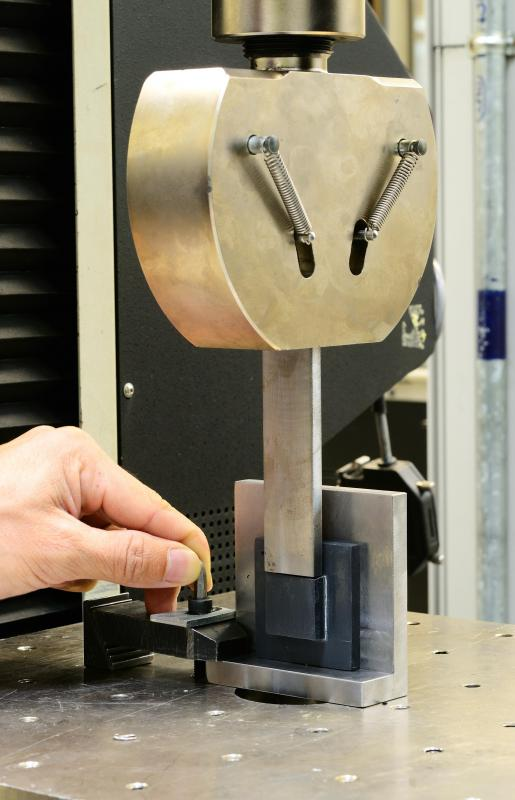# What is Ultimate Tensile Strength?

Jennifer Leigh

Ultimate tensile strength (UTS) is the maximum resistance that a material has to being fractured, and it is measured utilizing stress tests. A stress test on a material will provide data that can be plotted to form the material's load-versus-elongation curve and its stress-versus-strain curve. When looking at a material's stress-versus-strain curve, its ultimate tensile strength is the highest point on the curve. It often occurs before the breaking point because the material might crack or become weakened before it breaks. A material's ultimate tensile strength is often used for quality control purposes.Where a tensile bar breaks helps determine a material's ultimate strength.

Stress tests are fundamental in engineering processes where a material's load capacity is of importance, such as in construction. These tests are useful for determining a material's tensile strength, yield strength, elasticity, elastic limit and proportional limit. The UTS test measures load and elongation as more stress is applied over a specified period of time.Ultimate tensile strength is tested using pulling forces, usually administered by a machine that has been specially calibrated, to test a material's strength.Get started

UTS measurements can be found for a variety of materials, including metals and plastics, and new materials are often developed with higher UTS measurements. The ultimate tensile strength of a material is calculated by dividing the amount of the load at its failure point by the original area, which is expressed in pounds per square inch or kilograms per square centimeter, depending on which system of measurement is being used. The test can be set up so that the testing machine pulls the material apart at a rate of 0.2-20.0 inches (0.51-50.8 cm) per minute. This wide range of rates can affect the results of the UTS test, so certain industry standards must be followed when these tests are performed.

When it is under stress, material can become permanently damaged far before the breaking point. Ultimate tensile strength is often used for quality control purposes because it is easily reproducible compared to many other engineering tests, but it is not often used in the design of components because it is not necessarily representative of the highest amount of stress that the material can handle.

## You might also Like